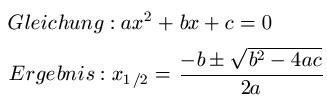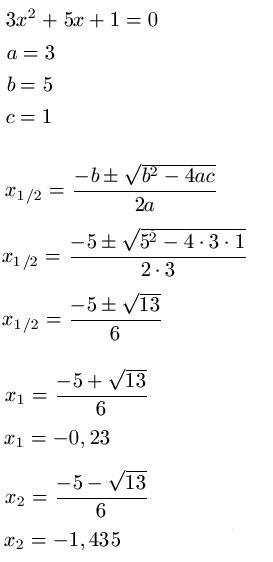# How to calculate a b c

## ABC formula

The ABC formula - often also called the midnight formula - is what we deal with in this math article. We will explain to you why you need the ABC formula and how you can use it during exercises.Everyone should be able to remember equations. An example: 5 + 3 = x. The solution x = 8 can be seen so quickly. By transforming equations, you can also solve complex equations. But what do you do when an equation like ax2 + bx + c = 0 exists? The answer is: use the ABC formula. And how exactly this works is covered in the following section. Before doing this, however, a few examples of quadratic equations, as many students quickly lose this:

• 5x2 + 3x + 2 = 0
• 1x2 + 7x + 3 = 2
• 87x2 - 3x + 1 = 0
Show:

### Apply the ABC formula

The ABC formula is very similar to the PQ formula and is used to solve quadratic equations. If you calculate correctly, you will get the same result with both formulas. The general formula and solution now follow, and then we turn to an example.Method:

To solve a quadratic equation using the ABC formula, we perform the following steps.

• We write down the starting equation
• We convert the equation so that there is only one zero (0) on one side.
• We find out a, b and c
• We put a, b and c in the formula
• We calculate the solution with a "+" in front of the root
• We calculate the solution with a "-" in front of the root

Example:

The equation 3x2 + 5x + 1 = 0 should be solved with the ABC formula.

Solution: From the equation we can see that a = 3, b = 5 and c = 1. We put these values ​​in the ABC formula and use them to calculate the solution.